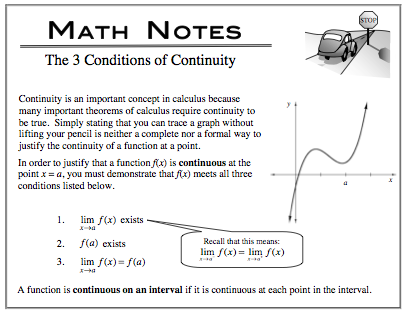### Home > CALC > Chapter 4 > Lesson 4.1.3 > Problem4-39

4-39.

Given the function $f ( x ) = \left\{ \begin{array} { l l } { 2 x ^ { 2 } - 4 \text { for } x \leq 3 } \\ { - 2 x - 5 \text { for } x > 3 } \end{array} \right.$ find:

1. $\lim\limits_ { x \rightarrow 3 ^ { + } } f ( x )$

Notice that the boundary point is $x = 3$. Which piece is to the right of that boundary point?

$\lim\limits_ { x \rightarrow 3 ^ { - } } f ( x )$

Which piece of $f(x)$ is to the left of the boundary point?

1. What do your results above tell you about $f(x)$?Do not claim continuity (or discontinuity) without accounting for all three conditions.